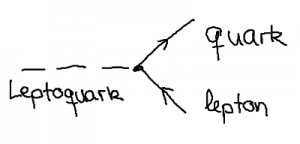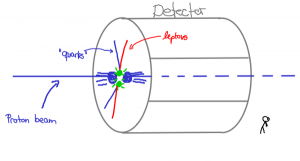Categories
Pages
-Are there leptoquarks?

July 16th, 2014 | byLeptoquarks are interesting objects: These (so far) hypothetical particles are able to connect a single Lepton and a single quark in one interaction. For triggering such an interaction, leptoquarks have to carry both lepton number (such as leptons) and baryon quantum number (such as quarks). Hence the name: Lept-o-quark.

In the Standard Model of particle physics, there are several reasons, why there is no such interaction: There is no particle that carries both lepton and quark numbers. With the observed particles (that are part of the Standard model), it is for mathematical reasons not possible to write down such an interaction.
However, these particles are theoretically very appealing: They appear if one wants to have a grand unified theory (GUT) which combines all interactions of the standard model into one. Also, in Supersymmetry with R-parity violation (R parity is that what keeps the supersymmetric dark matter stable), these particles can appear.

A recent publication of CMS  has set new limits on the mass of such particles. They investigate the hypothetical production and decays at the LHC: One assumes that these particles are produced in pairs. These two leptoquarks are then unstable and decay into a lepton and a quark, so in total two leptons and two quarks. And this is the channel where one can search for these particle: Events at the LHC that contain two leptons and two quarks.
This specific paper concentrates on the search for the first generation leptoquarks by  looking for events with two quarks and two electrons, or events with one electron, one electron-neutrino and two quarks:In both of these channels, an excess of around 2.4 and 2.6 sigma was seen for a leptoquark mass of around 650 GeV (almost 4 times as heavy as the heaviest quark, the top quark). Excess means, that one compares several quantities like energy and momenta of the quarks and electrons with the Standard model prediction. If the  observed number of quarks and electrons is, within statistical uncertainties, the same as the predicted one, then theory and data coincide. But if there are leptoquarks in nature, then one would actually see more quarks and electrons at that point. Hence the name “excess”.

Well, 2.6 sigma is not very much for particle physicists. A discovery in particle physics has to be made with 5 Sigma, that means that there should only be a 1 in roughly 2 million chance, that the excess one sees is due to statistical fluctuation.

Also, the distribution of energy and momenta of the electrons and quarks does not really fit to what one would expect from such a 650 GeV leptoquark in theory. A leptoquark would predict a quite concentrated, peaky energy distribution of the electrons and quarks, while the observed energy distributions are much broader.

So this is definitely something one should watch (and leptoquarks are cool, anyway), but nothing definite can be said with the current amount of data.